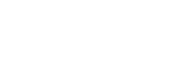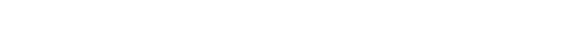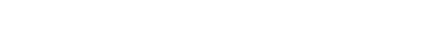Press "Enter" to skip to content

loss函数可以自定义

## 回归任务中的loss主要包括L2范数：L2损失表示了预测值与目标值之间差值的平方和然后开更方，L2表示的是欧几里得距离。MSE和L2的曲线走势都一样。区别在于一个是求的平均np.mean()，一个是求的更方np.sqrt()tensorflow和keras代码体现：

```#tensorflow
tf.losses.mean_squared_error(
labels,
predictions,
weights=1.0,
scope=None,
loss_collection=tf.GraphKeys.LOSSES,
reduction=Reduction.SUM_BY_NONZERO_WEIGHTS
)
tf.metrics.mean_squared_error(
labels,
predictions,
weights=None,
metrics_collections=None,
updates_collections=None,
name=None
)

#keras
mean_squared_error(y_true, y_pred)```L1范数：L1表示了预测值与目标值之间差值的绝对值，L1也叫做曼哈顿距离MAE和L1的区别在于一个求了均值np.mean()，一个没有求np.sum()。2者的曲线走势也是完全一致的。tensorflow和keras代码体现：

```#tensorflow
tf.metrics.mean_absolute_error(
labels,
predictions,
weights=None,
metrics_collections=None,
updates_collections=None,
name=None
)
#keras
mean_absolute_error(y_true, y_pred)```

MSE，MAE对比：MAE损失对于局外点更鲁棒，但它的导数不连续使得寻找最优解的过程低效；MSE损失对于局外点敏感，但在优化过程中更为稳定和准确。

zhuanlan.zhihu.com/p/58883095

## 1. 定义或者或者